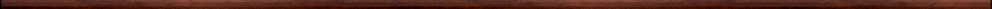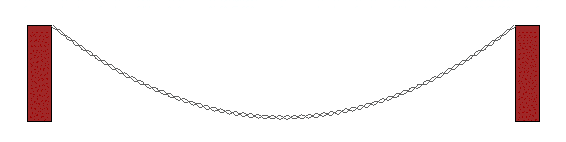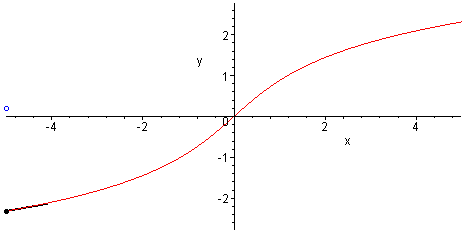Maple worksheets on hyperbolic functionsMore calculus topics:

They are all compatible with Classic Worksheet Maple 10.Hyperbolic functions - hyperbolic.mws

• Definition of the hyperbolic sine and cosine functions.
• Two points on the graph of sinh and cosh.
• The hyperbolic functions: tanh, sech, cosech, coth and their graphs.
• Some identities involving hyperbolic functions.
• Geometrical interpretation of the identity: cosh(x)^2-sinh(x)^2=1.
• The derivatives of the hyperbolic functions.Inverse hyperbolic functions - invhyp.mws

• The functions arcsinh, arccosh, arctanh, arcsech, arccsch, arccoth.
• Expressing the inverse hyperbolic functions in terms of the natural logarithm function.
• The derivatives of the inverse hyperbolic functions.

Integrals involving inverse hyperbolic functions - hypint.mws

• An integral involving arcsinh.
• An integral involving arccosh.
• An integral involving arcsech.
• An integral involving arccsch.
• Some other integrals involving inverse hyperbolic functions.

The curve formed by a uniform hanging chain - catenary.mws

• The derivation of the equation of the curve formed by a uniform hanging chain or cable.
• Comparison of catenary with parabola

Hyperbolic sectors and arcs  - hypsect.mws

• The area of a hyperbolic sector.
• Arc length along a parametric curve (including a rectangular hyperbola).

Top of page

Main index